Add two fractions by entering them below. You can optionally add a whole number for a mixed fraction as well.

Fraction One:
+
Fraction Two:

## Result as a Fraction:

1 / 3+1 / 4=7 / 12

## Result as a Decimal:

1 / 3+1 / 4=0.58333333333333

### Steps to Solve Expression with Fractions

Find the least common denominator so that all of the denominators match.
The least common denominator of 3 and 4 is 12
Find the multiple for each fraction that can be multiplied by the denominator to get the least common denominator.
Find the multiple by dividing the least common denominator by each fraction's denominator.
Fraction 1 Multiple: 12 ÷ 3 = 4
Fraction 2 Multiple: 12 ÷ 4 = 3
Multiply each fraction's numerator and denominator by the multiple.
1 × 4 / 3 × 4+1 × 3 / 4 × 3=4 / 12+3 / 12
Now that the denominators match, add the numerators
4 + 3 / 12=7 / 12
Reduce the fraction by finding the greatest common factor (GCF) of the numerator and denominator.
GCF of 7 and 12 = 1
Divide the numerator and denominator by the greatest common factor (1).
7 ÷ 1 / 12 ÷ 1=7 / 12
Find the decimal by dividing the numerator by the denominator.
7 / 12=7÷12=0.58333333333333
Learn how we calculated this below

A fraction is a numerical value that is not a whole number, and it generally represents a portion of a whole (like one slice of a pizza).

Adding fractions is a bit different from adding regular whole numbers, but you can use a few methods to do it.

The first method for adding fractions is to use a formula.

a / b + c / d = ad + bc / bd

To add two fractions, complete the following:

1. Multiply the numerator of the first fraction a with the denominator of the second fraction d.
2. Multiply the denominator of the first fraction b with the numerator of the second fraction c.
3. Add the products from step 1 and step 2 (ad + bc).
4. Divide the sum from step 3 (ad + bc) by the product of the denominators of both fractions (b and d).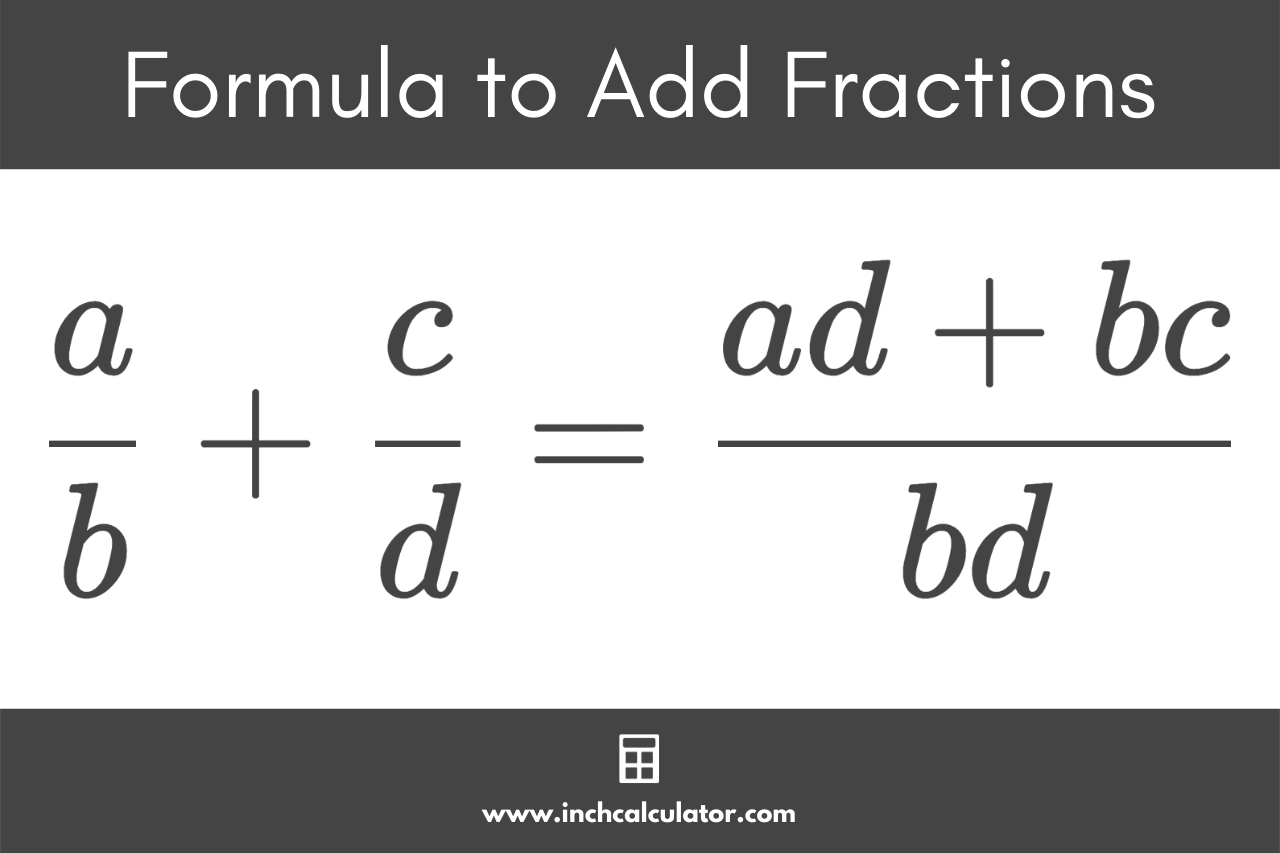By multiplying the denominators together, the formula ensures that they both match and are both raised to a common denominator.

The numerators are also multiplied using the same multiple as the denominator, resulting in an equivalent fraction.

Then, the resulting numerators can simply be added together.

You can put this formula to practice and add two fractions in three easy steps.

Follow along with this example showing how to add 1 / 3 and 1 / 4.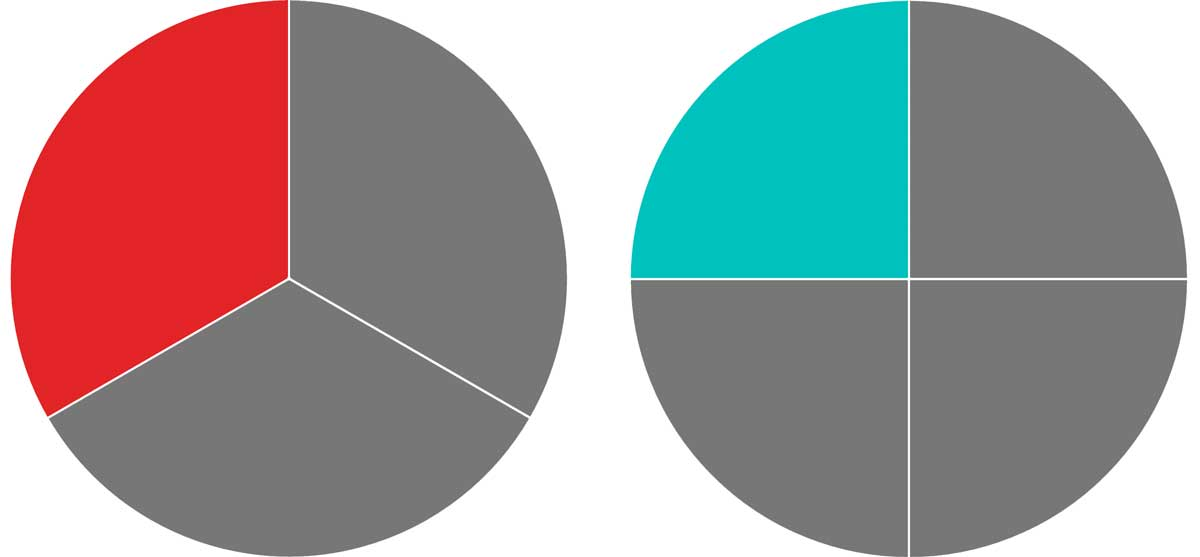### Step One: Convert to Fractions with the LCD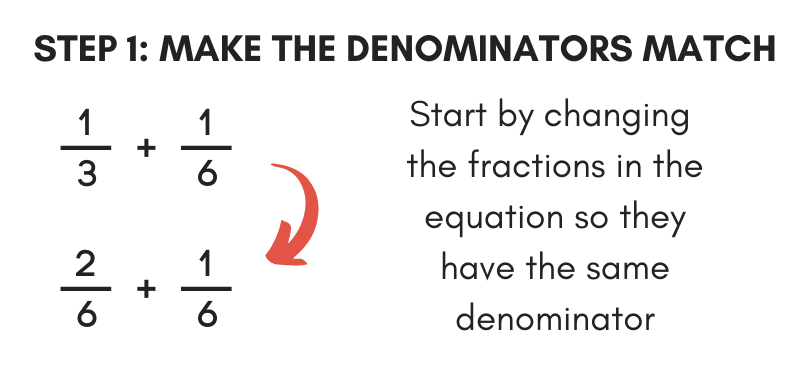When adding fractions, the first step is to ensure that they have the same denominator. Convert each fraction to an equivalent fraction with a matching denominator to the other. To do this, you need to find the lowest common denominator (LCD), which is the smallest number that both denominators can divide into evenly.

#### Find the Multiplier

Once you have found the lowest common denominator, you will then find what number, or multiple, you need to multiply each fraction to make the denominators match. To do this, divide the LCD by the denominator of each fraction.

#### Multiply by the Multiplier

Then, multiply both the top and bottom number of the fraction by the multiple to find the equivalent fractions with matching denominators. You can also use an equivalent fractions calculator to find one with a matching denominator.

For example, let’s convert the fractions 1 / 3 and 1 / 4 to fractions with the same denominator.

Find the least common denominator. The least common denominator of 3 and 4 is 12.

Find the multiple for 1 / 3
multiple = lcd ÷ denominator
multiple = 12 ÷ 3 = 4

Find the equivalent fraction of 1 / 3 using the multiple 4
1 / 3 = 1 × 4 / 3 × 4
1 / 3 = 4 / 12

Find the multiple for 1 / 4
multiple = lcd ÷ denominator
multiple = 12 ÷ 4 = 3

Find the equivalent fraction of 1 / 4using the multiple 3
1 / 4 = 1 × 3 / 4 × 3
1 / 4 = 3 / 12

Thus, the equivalent fractions of 1 / 3 and 1 / 4 are 4 / 12 and 3 / 12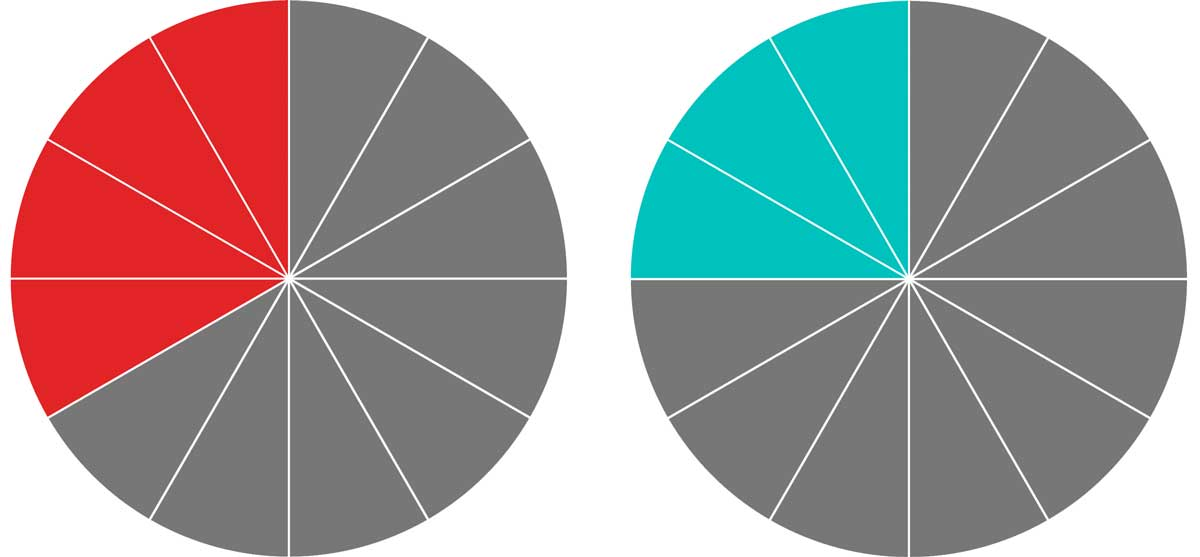### Step Two: Add the Numerators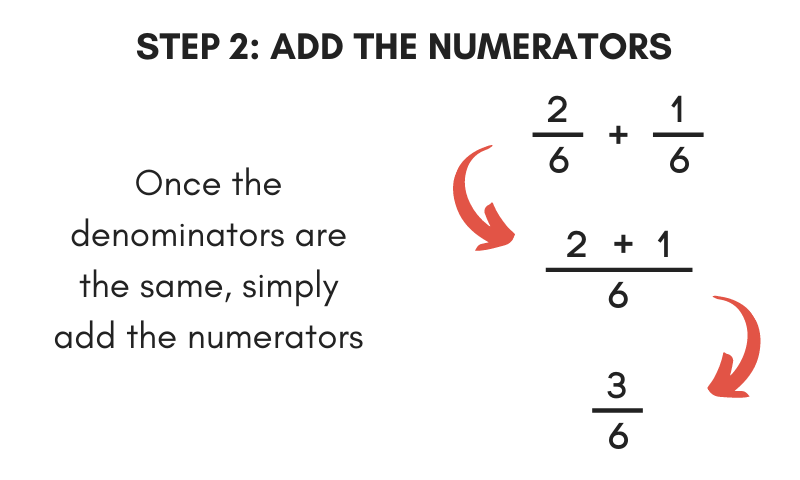Once the bottom numbers of each fraction match, add the top numbers of each together to find the result.

Simply add the numerators together and put them over the common denominator.

For example, let’s continue the previous example and add 4 / 12 & 3 / 12.

4 / 12 + 3 / 12 = 4 + 3 / 12
4 / 12 + 3 / 12 = 7 / 12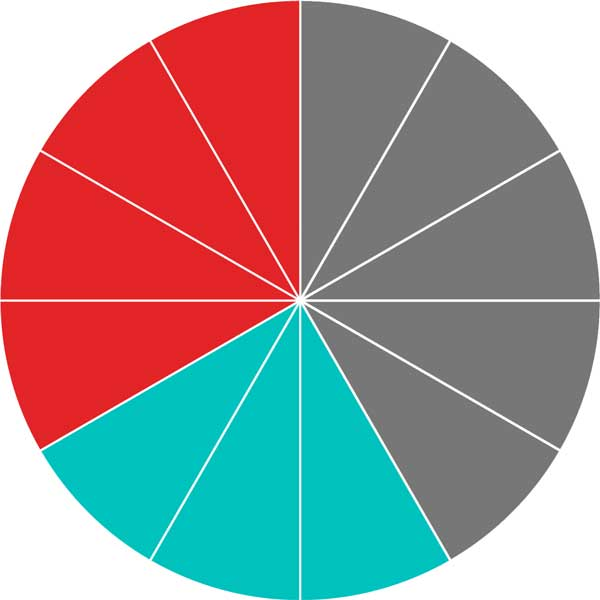### Step Three: Simplify the Fraction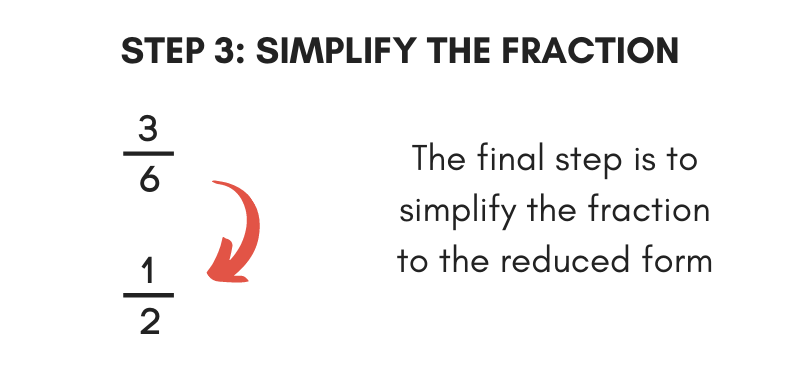The final addition step is to reduce the resulting fraction to its simplest form. Start by finding the greatest common factor of the top and bottom parts of the result.

Then, divide both the top and bottom numbers by the greatest common factor to reduce it to the lowest terms. An even easier way to reduce is to use our fraction simplifier.

We also have a calculator to multiply fractions or divide fractions.

## How to Add Mixed Numbers

A mixed number is a number with both a whole number and a proper fraction. The process of adding mixed numbers is nearly the same as adding a normal fraction with one more step.

The first thing to do when adding mixed numbers is to make the fractions improper fractions by removing the whole number and moving it to the numerator. You can use a mixed number to fraction calculator or follow the steps below.

To do this, multiply the whole number by the denominator, then add this to the fraction numerator.

For example, let’s convert the mixed number 1 2 / 3 to an improper fraction.

Multiply the whole number by the denominator.

1 × 3 = 3

Next, add the result to the numerator.

3 + 2 = 5

Finally, rewrite the fraction.

1 2 / 3 = 5 / 3

## How to Add Negative Fractions

A negative fraction is one with a minus sign either in front of the fraction or in front of the numerator or denominator.

a / b = -a / b = a / -b

Negative fractions have only one negative sign. So, if both the numerator and the denominator have a negative sign, then the fraction is actually positive.

When adding negative fractions, it’s easiest to put the sign on the numerator, which allows you to use the fraction addition formula above.

### Can you add fractions and decimals?

Yes, you can add fractions and decimals, but you need to convert one of the values to a format that matches the other. For example, if you want to add the fraction 1/2 to the decimal 0.25, you could convert 1/2 to 0.5 or convert 0.25 to 1/4.

### What are the rules to add fractions?

The four rules to add fractions are:

1. Find a common denominator.
2. Convert the fractions so that they have the same denominator.